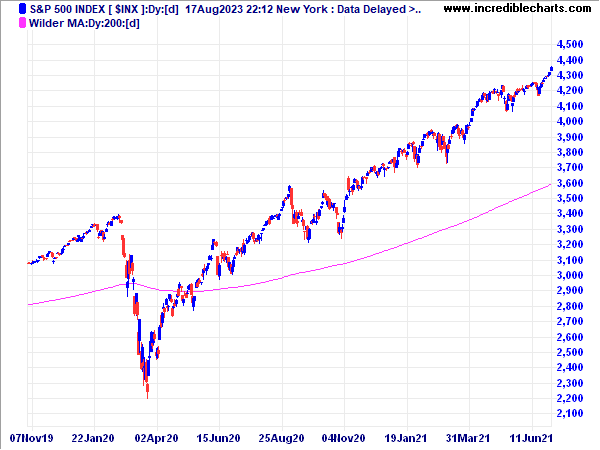# Wilder Moving Average

A number of popular indicators, including Relative Strength Index (RSI), Average True Range (ATR) and Directional Movement were developed by J. Welles Wilder and introduced in his 1978 book: New Concepts in Technical Trading Systems. Users should beware that Wilder does not use the standard exponential moving average formula. This can have significant impact when selecting suitable time periods for his indicators.## Welles Wilder's Moving Average Formula

The standard exponential moving average formula converts the time period to a fraction using the formula EMA% = 2/(n + 1) where n is the number of days. For example, the EMA% for 14 days is 2/(14 days +1) = 13.3%. Wilder, however, uses an EMA% of 1/14 which equals 7.1%. This equates to a 27-day exponential moving average using the standard formula.

## Welles Wilder's Indicators

Indicators affected are:

## Indicator Time Frames

We recommend that users try shorter time periods when using one of the above indicators. For example, if you are tracking a 30-day cycle you would normally select a 15-day Indicator Time Period. With the RSI, adjust the time period as follows:

RSI time period = (n + 1) / 2 = (15 + 1) / 2 = 8 days